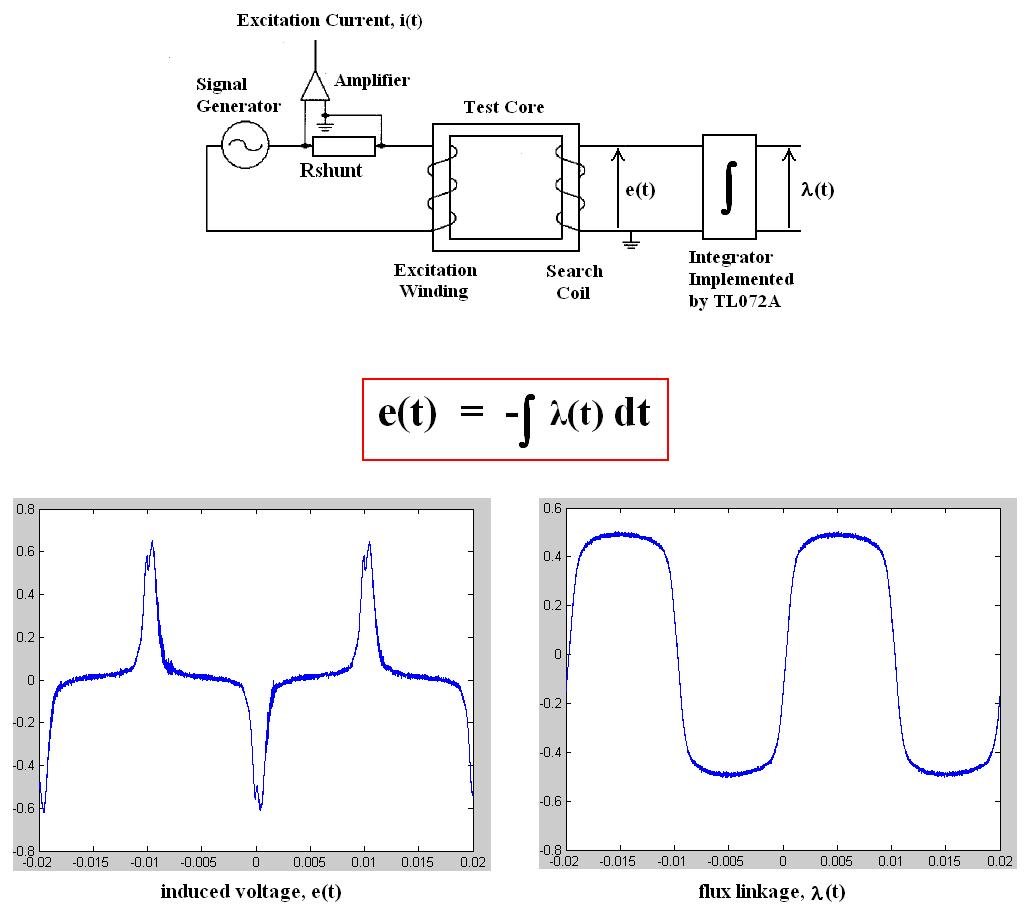# How to perform integration on this measured data?

Status
Not open for further replies.

#### powersys

I measured the induced voltage e(t), as well as the flux linkage λ(t) (see the measured waveforms below). I wish to obtain the flux linkage by performing 'software' numerical integration over the measured e(t). Anyone know the code to do so in MATLAB? Thanks.Use C code for Simpson or trapezoidal rule.If u have Mathematica then there is a routine to do so,otherwise writing a simple C code is gud one.
Use Numerical recipies in C/C++.

atul_microwaves_antenna said:
Use C code for Simpson or trapezoidal rule.If u have Mathematica then there is a routine to do so,otherwise writing a simple C code is gud one.
Use Numerical recipies in C/C++.
I don't think it's that straight-forward... using the standard routine will give the total area.. Pls advise...

Status
Not open for further replies.